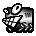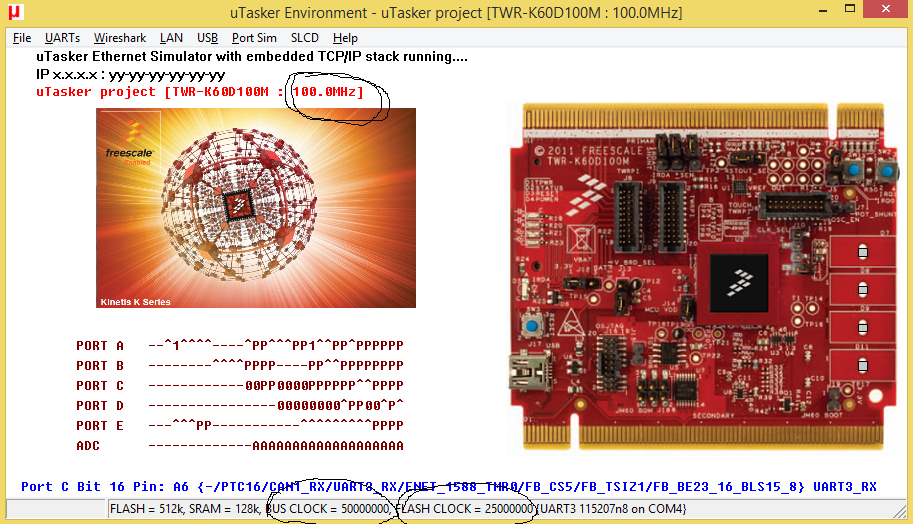## K60 hangs waiting for MCG_S_IREFST

cancel
Showing results for
Did you mean:

## K60 hangs waiting for MCG_S_IREFST

630 ViewsContributor IV

Hi everyone,

I'm using a k60 that is supplied with an external 50MHz clock.  I used Processor Expert to generate code to set up the clocks, but the code hangs while it's waiting for the FLL reference clock to be supplied by the external reference clock.  I've looked through the code and I can't find anything wrong.  Here's the code I'm using:

/*** ### MK60DN256VLQ10 "Cpu" init code ... ***/

/*** PE initialization code after reset ***/

/* System clock initialization */

/* SIM_CLKDIV1: OUTDIV1=0,OUTDIV2=1,OUTDIV3=3,OUTDIV4=3,??=0,??=0,??=0,??=0,??=0,??=0,??=0,??=0,??=0,??=0,??=0,??=0,??=0,??=0,??=0,??=0 */

SIM_CLKDIV1 = SIM_CLKDIV1_OUTDIV1(0x00) |

SIM_CLKDIV1_OUTDIV2(0x01) |

SIM_CLKDIV1_OUTDIV3(0x03) |

SIM_CLKDIV1_OUTDIV4(0x03); /* Set the system prescalers to safe value */

/* SIM_SCGC5: PORTA=1 */

SIM_SCGC5 |= SIM_SCGC5_PORTA_MASK;   /* Enable clock gate for ports to enable pin routing */

if ((PMC_REGSC & PMC_REGSC_ACKISO_MASK) != 0x0U) {

/* PMC_REGSC: ACKISO=1 */

PMC_REGSC |= PMC_REGSC_ACKISO_MASK; /* Release IO pads after wakeup from VLLS mode. */

}

/* SIM_CLKDIV1: OUTDIV1=0,OUTDIV2=1,OUTDIV3=1,OUTDIV4=3,??=0,??=0,??=0,??=0,??=0,??=0,??=0,??=0,??=0,??=0,??=0,??=0,??=0,??=0,??=0,??=0 */

SIM_CLKDIV1 = SIM_CLKDIV1_OUTDIV1(0x00) |

SIM_CLKDIV1_OUTDIV2(0x01) |

SIM_CLKDIV1_OUTDIV3(0x01) |

SIM_CLKDIV1_OUTDIV4(0x03); /* Update system prescalers */

/* SIM_SOPT2: PLLFLLSEL=0 */

SIM_SOPT2 &= (uint32_t)~(uint32_t)(SIM_SOPT2_PLLFLLSEL_MASK); /* Select FLL as a clock source for various peripherals */

/* SIM_SOPT1: OSC32KSEL=3 */

SIM_SOPT1 |= SIM_SOPT1_OSC32KSEL(0x03); /* LPO 1kHz oscillator drives 32 kHz clock for various peripherals */

/* PORTA_PCR18: ISF=0,MUX=0 */

/* Switch to FBE Mode */

/* MCG_C2: LOCRE0=0,??=0,RANGE0=2,HGO0=0,EREFS0=0,LP=0,IRCS=0 */

MCG_C2 = MCG_C2_RANGE0(0x02);

/* OSC_CR: ERCLKEN=1,??=0,EREFSTEN=0,??=0,SC2P=0,SC4P=0,SC8P=0,SC16P=0 */

/* MCG_C7: OSCSEL=0 */

/* MCG_C1: CLKS=2,FRDIV=5,IREFS=0,IRCLKEN=1,IREFSTEN=0 */

MCG_C1 = (MCG_C1_CLKS(0x02) | MCG_C1_FRDIV(0x05) | MCG_C1_IRCLKEN_MASK);

/* MCG_C4: DMX32=0,DRST_DRS=0 */

/* MCG_C5: ??=0,PLLCLKEN0=0,PLLSTEN0=0,PRDIV0=0x0F */

MCG_C5 = MCG_C5_PRDIV0(0x0F);

/* MCG_C6: LOLIE0=0,PLLS=0,CME0=0,VDIV0=8 */

MCG_C6 = MCG_C6_VDIV0(0x08);

while((MCG_S & MCG_S_IREFST_MASK) != 0x00U) { /* Check that the source of the FLL reference clock is the external reference clock. */     <<<<<-------------    THIS LINE HANGS FOREVER

}

while((MCG_S & 0x0CU) != 0x08U) {    /* Wait until external reference clock is selected as MCG output */

}

/* Switch to PBE Mode */

/* MCG_C6: LOLIE0=0,PLLS=1,CME0=0,VDIV0=8 */

while((MCG_S & 0x0CU) != 0x08U) {    /* Wait until external reference clock is selected as MCG output */

}

while((MCG_S & MCG_S_LOCK0_MASK) == 0x00U) { /* Wait until locked */

}

/* Switch to PEE Mode */

/* MCG_C1: CLKS=0,FRDIV=5,IREFS=0,IRCLKEN=1,IREFSTEN=0 */

MCG_C1 = (MCG_C1_CLKS(0x00) | MCG_C1_FRDIV(0x05) | MCG_C1_IRCLKEN_MASK);

while((MCG_S & 0x0CU) != 0x0CU) {    /* Wait until output of the PLL is selected */

}

/*** End of PE initialization code after reset ***/

Labels (2)

• ### Kinetis K Series MCUs

Tags (1)
7 Replies
127 ViewsContributor IV

Hi everyone, thanks for responding to my question.  I poked around the hardware, and found that there was an issue preventing the external clock from starting.  Once that was sorted out, the code generated by PE worked correctly.

127 ViewsSpecialist V

Hi Marlon

To quote Dr Sheldon Cooper:;-)

Regards

Mark

127 ViewsNXP Employee

Hi Smith,

I think the MCG initialization code looks not right. If the input clock is 50MHz, then the configuration here clearly is not right.  Are you sure this is generated with PE?

PLL input clock for K60 100MHz part should be in 2-4MHz range.

MCG_C5 = MCG_C5_PRDIV0(0x0F);

/* MCG_C6: LOLIE0=0,PLLS=0,CME0=0,VDIV0=8 */

MCG_C6 = MCG_C6_VDIV0(0x08);

A working configuration should be like below.

MCG_C5 = MCG_C5_PRDIV0(0x18); //divide by 25 to get 2MHz

/* MCG_C6: LOLIE0=0,PLLS=0,CME0=0,VDIV0=8 */

MCG_C6 = MCG_C6_VDIV0(0x1C);     //multiply with 48 to get 96MHz core clock

Hao

127 ViewsContributor IV

I used PE myself to generate the code.  The external reference clock is 50 MHz, so it looks like PE is setting PRDIV0 to 0x0F, so the 50 MHz clock is divided by 16 to give 3.125 MHz.  Then it's setting VDIV0 to 0x08, which multiplies the 3.125 MHz by 32, to give a final value of 100 MHz.

Does this seem correct?

127 ViewsSpecialist V

Marlon

I see no problem with your input divider.

Did you check that the 50MHz clock is really present (by measuring it)?

Regards

Mark

127 ViewsSpecialist V

Marlon

It may be that your 50MHz is missing at the input since without it the switch-over won't take place.

Check jumpers (assuming it is a tower kit - recommended jumper settings are on page 9 of http://www.utasker.com/docs/KINETIS/uTaskerV1.4_Kinetis_demo.pdf )

For a discussion of the MCG, modes and some practical details see http://www.utasker.com/kinetis/MCG.html

I think that your code is aiming at setting 100MHz core. Below is the same setting which the uTasker K60 tower kit simulator shows - it uses this code (as comparison).

```    MCG_C2 = (MCG_C2_RANGE_8M_32M | MCG_C2_LOCRE0);                      // select external clock source (with reset on clock loss)
MCG_C1 = (MCG_C1_CLKS_EXTERN_CLK | MCG_C1_FRDIV_1024);               // switch to external input clock (the FLL input clock is set to as close to its input range as possible, although this is not absolutely necessary since the FLL will not be used)
while ((MCG_S & MCG_S_IREFST) != 0) {                                // loop until the FLL source is no longer the internal reference clock
}
while ((MCG_S & MCG_S_CLKST_MASK) != MCG_S_CLKST_EXTERN_CLK) {       // loop until the external reference clock source is valid
}
MCG_C5 = ((CLOCK_DIV - 1) | MCG_C5_PLLSTEN0);                        // now move from state FEE to state PBE (or FBE) PLL remains enabled in normal stop modes
MCG_C6 = ((CLOCK_MUL - MCG_C6_VDIV0_LOWEST) | MCG_C6_PLLS);
while ((MCG_S & MCG_S_PLLST) == 0) {                                 // loop until the PLLS clock source becomes valid
}
while ((MCG_S & MCG_S_LOCK) == 0) {                                  // loop until PLL locks
}
SIM_CLKDIV1 = (((SYSTEM_CLOCK_DIVIDE - 1) << 28) | ((BUS_CLOCK_DIVIDE - 1) << 24) | ((FLEX_CLOCK_DIVIDE - 1) << 20) | ((FLASH_CLOCK_DIVIDE - 1) << 16)); // prepare bus clock divides
MCG_C1 = (MCG_C1_CLKS_PLL_FLL | MCG_C1_FRDIV_1024);                  // finally move from PBE to PEE mode - switch to PLL clock
while ((MCG_S & MCG_S_CLKST_MASK) != MCG_S_CLKST_PLL) {              // loop until the PLL clock is selected
}```

The input to achieve this is:

```    #define CLOCK_DIV            16                                      // input must be divided to 2MHz..4MHz range (/1 to /25 possible)
#define CLOCK_MUL            32                                      // the PLL multiplication factor to achieve operating frequency of 100MHz (x24 to x55 possible)

#define SYSTEM_CLOCK_DIVIDE  1                                       // 1 to 16 - usually 1
#define BUS_CLOCK_DIVIDE     2                                       // 1 to 16
#define FLEX_CLOCK_DIVIDE    2                                       // 1 to 16
#define FLASH_CLOCK_DIVIDE   4                                       // 1 to 16```Regards

Mark

For the complete "out-of-the-box" Kinetis experience and faster time to market

:smileyinfo: Out-of-the-box support for 46 Kinetis boards and 10 IDEs (460 combinations from a single code source with no porting required)

127 ViewsContributor IV

Hi Mark, thanks for the reply.  You're right, my code is aiming for a 100MHz core clock.  It's not a tower kit, but now that you mention it I should check to make sure the external clock is actually running properly, as this is a new hardware prototype.  Thanks for the code, I'll double check that against mine as well.

Marlon